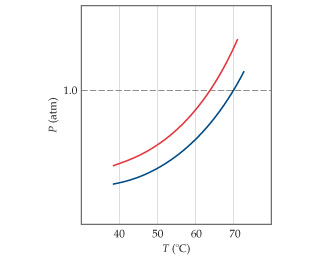# Problem: The following diagram shows the vapor pressure curves of a volatile solvent and a solution of that solvent containing a nonvolatile solute.What are the normal boiling points of the solvent and the solution?

###### FREE Expert Solution

We are being asked to determine the normal boiling points of the solvent and solution.

Recall that the normal boiling point of a liquid is the temperature at which its vapor pressure is equal to 1 atm.

99% (150 ratings)###### Problem Details

The following diagram shows the vapor pressure curves of a volatile solvent and a solution of that solvent containing a nonvolatile solute.What are the normal boiling points of the solvent and the solution?

Frequently Asked Questions

What scientific concept do you need to know in order to solve this problem?

Our tutors have indicated that to solve this problem you will need to apply the Boiling Point Elevation concept. If you need more Boiling Point Elevation practice, you can also practice Boiling Point Elevation practice problems.

What professor is this problem relevant for?

Based on our data, we think this problem is relevant for Professor Reeves' class at UNCW.